Previous Article in Journal
Sensor Mania! The Internet of Things, Wearable Computing, Objective Metrics, and the Quantified Self 2.0
Article

Range-Free Localization in Wireless Sensor Networks with Neural Network Ensembles

Department of Computer Science and Engineering, New Mexico Institute of Mining and Technology, 801 Leroy Place, Socorro, NM 87801, USA
*
Author to whom correspondence should be addressed.
J. Sens. Actuator Netw. 2012, 1(3), 254-271; https://doi.org/10.3390/jsan1030254
Received: 19 September 2012 / Revised: 8 November 2012 / Accepted: 15 November 2012 / Published: 28 November 2012

Abstract

In wireless sensor networks (WSNs), the location information of sensor nodes are important for implementing other network applications. In this paper, we propose a range-free Localization algorithm based on Neural Network Ensembles (LNNE). The location of a sensor node is estimated by LNNE solely based on the connectivity information of the WSN. Through simulation study, the performance of LNNE is compared with that of two well-known range-free localization algorithms, Centroid and DV-Hop, and a single neural network based localization algorithm, LSNN. The experimental results demonstrate that LNNE consistently outperforms other three algorithms in localization accuracy. An enhanced mass spring optimization (EMSO) algorithm is also proposed to further improve the performance of LNNE by utilizing the location information of neighboring beacon and unknown nodes.

1. Introduction

Wireless sensor networks (WSNs) are the collections of spatially distributed autonomous inexpensive sensors with limited resources that are deployed in two or three dimensional geographic domains and cooperatively monitor physical or environmental conditions . In WSNs, the geographic location information of sensor nodes is important for many WSN applications such as network management, monitoring, target tracking, geographic routing, etc. Although the position of a sensor can be easily acquired with an integrated Global Positioning System (GPS) module, it is extremely expensive to have GPS modules equipped on all the sensor nodes in WSNs. An alternative solution is that only a limited number of nodes called beacon nodes or anchor nodes can equip GPS modules to accurately acquire their positions . The beacon nodes will then assist the positioning of other nodes without GPS modules (called unknown nodes) based on a localization algorithm.
The localization algorithms proposed for WSNs can be divided into range-based and range-free categories according to the type of input data. The range-based algorithms compute the positions of unknown nodes based on the assumption that the absolute distance between an unknown node and a beacon node can be estimated from ranging measurements such as Received Signal Strength Indication (RSSI) [3,4], Time of Arrival (ToA) , Time Difference of Arrival (TDoA) [6,7], or Angle of Arrival (AoA) [5,8,9]. However, to achieve accurate measurements, normally extra special and expensive ranging hardware are required. Measurements such as RSSI can utilize the existing device in sensor node but are not accurate. On the other hand, range-free algorithms, such as Centroid , APIT , SeRLoc , Multidimensional Scaling , Ad-Hoc Positioning System (APS) , only use the connectivity or proximity information to localize the unknown nodes. The range-free algorithms generally require only simple operations and do not need additional hardware, which makes them attractive to be applied for WSNs.
Recently, a number of machine learning based localization techniques have been proposed [15,16,17,18]. Instead of using the geometric properties to find the unknown nodes’ locations, the machine learning based methods utilize the known locations of beacon nodes as training data to construct a prediction model for localization purpose. The learning algorithms employed to build the prediction model include Neural Networks (NN) [15,18] and Support Vector Machines (SVM) [16,17,19]. The input data to the learning algorithms can be signal strengths [16,18,19]) or hop-count information [15,17].
In this study, we propose to use the ensemble of neural networks to build the sensor position prediction model based on the hop-count information. An ensemble of multiple predictors has been shown, which has better performance than a single predictor in average [20,21,22]. Based on our study, we demonstrate that the localization accuracy can be significantly improved by using neural network ensemble (NNE) compared with a single NN based localizer.
The rest of this paper is organized as follows. Section 2 gives a brief introduction of previous works in localization for WSNs. In Section 3, we describe the proposed LNNE, a range-free localization algorithm based on neural network ensembles. Section 4 presents the simulation results. Finally, the conclusions are drawn in Section 5.

2. Related Work

In this section, we briefly review some related works on range-free and machine learning based localization algorithms.
Bulusu et al.  proposed the Centroid algorithm to estimate the nodes’ location. In the algorithm, the beacon nodes are placed in a grid configuration while an unknown node’s location is estimated as the centroid of the locations of all beacon nodes heard. The Centriod algorithm is simple and easy to implement but the localization accuracy heavily relies on the percentage of deployed beacon nodes.
Another well-known range-free localization algorithm is DV-Hop proposed by Niculescu and Nath . In DV-Hop, each beacon node computes the Euclidean distances to other beacon nodes and estimates its average hop length with the hop-count information. The unknown nodes then utilize the average hop length estimates to determine their distances to beacon nodes and apply lateration to calculate the location estimates.
Nguyen et al.  first applied machine learning technique to the sensor network localization problem. The signal strength measured by the sensor nodes were used to define the basis functions of a kernel-based learning algorithm, which was then applied to the localization of the unknown nodes. In , Shilton et al. also considered the localization in WSN as a regression problem with the received signal strengths as input. They applied a range of support vector regression (SVR) techniques and found that ε-SVR had the best performance.
Tran and Nguyen  proposed LSVM, a range-free localization algorithm based on SVM learning. The algorithm assumes that a sensor node may not communicate directly with a beacon node and only connectivity information is used for location estimation. A modified mass spring optimization (MMSO) algorithm was also proposed to further improve the estimation accuracy of LSVM.
A neural network based localization algorithm was proposed in . The RSS values between an unknown node and its adjacent beacon nodes were used as the input to the neural network. The trained neural network then mapped the RSS values to the estimated location of the unknown node. The approach requires that the unknown can hear from beacon nodes directly to measure the RSS values. Thus, the beacon nodes have to be placed regularly in a grid.
Chatterjee  developed a Fletcher–Reeves update-based conjugate gradient (CG) multilayered feed forward neural network for multihop connectivity-based localization in WSN. The method adopts the same assumptions as  and has better performance than LSVM. It has been shown in  that the neural network based localizer has better performance than LSVM and a diffusion-based method.
In this paper, we aim to improve the performance of single neural network based localizer of  by using the ensemble of neural networks.

3. Methods

In this section, we present the proposed localization algorithm based on NNEs. The algorithm adopts similar modest assumptions as [15,17]: (1) there are beacon nodes in the network; (2) a sensor node may not communicate with beacon nodes directly; (3) the localization algorithm only utilizes the connectivity information.

3.1. Network Model

We consider a large scale WSN with N nodes, S = {S1, S2, ..., SN }. All nodes in the network have the same transmission range r. The network is deployed in a 2-D geographical area of size D × D. We assume that there are M (M < N ) beacon nodes in the network that know their own locations. The remaining (N M ) unknown nodes can estimate their own locations by using a localization algorithm.We assume that each node can communicate with a beacon node through a multihop path as in [15,17].

3.2.1. System Architecture

In the LNNE system, two NNEs with the same architectures X -NNE and Y -NNE are used to estimate the x and y coordinates respectively. In the following, we use X -NNE as an example to illustrate the system.
The architecture of X-NNE is shown in Figure 1. The X -NNE consists of K component neural network where each component neural network is a three-layer feed forward neural network (FFNN) with nh nodes in the hidden layer. The number of nodes in the input layer, ni, is M , which is the same as the number of beacon nodes. The input of each node in this layer is the number of hops from a sensor node Si to the corresponding beacon nodes Sj, h(Si,Sj)(1 i N, 1 j M ). The number ofnodes in the output layer of each component network, no, is one, which is the estimated x coordinate.Each component neural network is trained with different initial weights connecting three layers. The final output of the NNE is the combination of the outputs of all component neural networks, which is computed by the combination module.
Figure 1. X-NNE architecture.
Figure 1. X-NNE architecture.Two combination rules are considered in this study:
(1) Mean rule: The output of the X-NNE is the average of the outputs of all component neural networks, which is defined aswhere XestSi is the estimated x coordinate, xestSi,k is the estimated x coordinate of kth component neural network, k = 1, 2, , K .
(2) Median rule: The output of the X -NNE is the median of the outputs of all component neural networks, i.e., XestSi= Median(xestSi,k), k = 1, 2, , K .

3.2.2. Component Neural Network

The component neural network used in the localization system is a three layer Fletcher–Reeves update-based conjugate gradient FFNN with M input nodes, nh hidden nodes, and one output node. In this study, we set the number of hidden nodes nh as round (). Each component neural network has the same architecture. Figure 2 shows an example of the kth component neural network for X -NNE.
Figure 2. A component neuralnetwork of X-NNE.
Figure 2. A component neuralnetwork of X-NNE.The input vector of each component neural network is HSi = [h(Si, S1), h(Si, S2), ..., h(Si, SM)], where h(Si, Sm)(1 m M ) is the hop-count length of the shortest path between sensor node Si and beacon node Sm. The output of the network is as followswhere vmn(1 m M, 1 n nh) are the weights connecting input layer to hidden layer, wn(1 n nh) are the weights connecting hidden layer to output layer, fh{•} and fo{•} are the activation functions for the hidden layer and the output layer respectively.
The connection weights of each component neural network have to be adjusted through a learning algorithm based on the training data. The training data set for X -NNE is {(HS1,xS1 ,k), (HS2,xS2 ,k), ..., (HSM,xSM,k)}, where xSm,k is the x-coordinate of the beacon node Sm(1 m M ). The back propagation (BP) method is the widely used learning algorithm thattries to minimize the error function of the network, which is defined asDuring the training process, the weights of the network are updated as follows:where α represents the learning constant that defines the step length of each iteration in the negative gradient direction. The weights of the network are adjusted iteratively until the error function converges to a minimum. The BP method is easy to implement but has a slow convergence speed.
The performance of the BP algorithm can be improved by allowing the learning rate change during the training process. The conjugate gradient algorithm is such a method to accelerate the convergence speed , which carries out the search along conjugate directions. The algorithm searches in the steepest descent direction first.The algorithm performs a linear search to determine the optimal distance to move along the current search directionThe next search is then performed in the conjugate direction of the previous directions. The algorithm determines the new search direction by combining the new steepest descent direction with the previoussearch direction: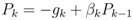where βk is the scaling constant. In the Fletcher–Reeves update , βk is computed as the ratio of the norm squared of the current gradient to the norm squared of the previous gradient.

3.2.3. Protocol

The protocol used in the LNNE system consists of four phases: info phase, training phase, advertisement phase, and localization phase.
Info phase: In this phase, each beacon node broadcasts a HELLO message to all other nodes in the network. A receiving node can obtain the hop-count information to the sending beacon node from the received HELLO message. The number of HELLO messages broadcasted in this phase is M .
Training phase: The training of the LNNE system is time and resource consuming. We assume that the training is conducted in a resource-rich head beacon node, which could be the base station or the sink node of the sensor network. In the beginning of this phase, each beacon node sends an INFO message to the head beacon node. The INFO message of the sending node contains the hop-count information to other beacon nodes gathered in the info phase and its true location. After the hop-count and location information of the beacon nodes are gathered, the head beacon node trains the NNEs. This phase requires the unicast delivery of M INFO messages.
Advertisement phase: The head beacon node broadcast the information of the trained NNEs to all the sensor nodes in the network. The size of the message depends on the number of component neural networks and the number of hidden nodes of each component neural network.
Localization phase: After receiving the information of the trained NNEs, each unknown node estimates its location with the hop-count information gathered in the info phase as input to the NNEs.

3.2.4. Refinement Algorithm

In , an algorithm modified from the mass-spring optimization (MSO) algorithm of  was proposed to improve the location estimation accuracy. The MSO algorithm considers each sensor node as a “mass” and adjusts a node’s location by using the “spring force” computed from its neighbors’ locations. The algorithm assumes that the distance between adjacent nodes is measurable. Since the LSVM algorithm of  assumes no knowledge on the true distance between two nodes, the transmission range is used in the Modified MSO (MMSO) algorithm.
The refinement of unknown node’s position of MMSO algorithm is done with the help of the neighboring beacon nodes. To further improve the performance, we propose an enhanced mass-spring optimization (EMSO) algorithm that utilizes the location information of both the neighboring beacon and unknown nodes.
We denote Φ(Si) = {Sj|h(Si, Sj) = 1, Dest(Si, Sj) > r, Sj B} as the set of beacon nodes that are 1-hop neighbors of sensor node Si with the distance to Si larger than the transmission range r, where B = {Si|Si S, 1 i M } is the set of beacon nodes, Dest(Si, Sj) is the distance between two sensor nodes Si and Sj based on their estimated locations. Ψ(Si) = {Sj|h(Si, Sj) = 1, Dest(Si, Sj) > r, Sj U} is denoted as the set of unknown nodes that are 1-hop neighbors of Si with the distance to Si larger than r, where U = {Si|Si S, M + 1 i N } is the set of unknown nodes. The number of nodes in Φ(Si) and Ψ(Si) are denoted as |Φ(Si)| and |Ψ(Si)|, respectively. Let N (Si) = Φ(Si) U Ψ(Si) be the set of all 1-hop neighbors of Si with the distance to Si larger than r.
To improve localization accuracy, we need to minimize the total energy of the system,, where E(Si) is the energy of a sensor node Si defined asIn the mass-spring system, the force on the sensor node Si pulled by a neighboring sensor node Sj is defined as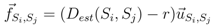whereis the unit vector from Si to Sj. When computing the total force applied on the sensor node Si pulled by all 1-hop neighboring nodes, we give a higher weight to the beacon node as the location of beacon node is known. The total force on Si is then defined aswhere wb= 1 and wu= 0.5 are the weights for neighboring beacon and unknown nodes, respectively. The x-dimension and y-dimension magnitude of the total force on Si are denoted as FX,Si and FY,Si.
The EMSO algorithm for improving the location estimation of all unknown nodes is illustrated in Algorithm 1. The algorithm minimizes the total energy Et by minimizing the local energy of each unknown node. The unknown node’s estimated location is adjusted if the new location reduces the local energy. The algorithm terminates when the maximum number of iterations T is reached. The EMSO algorithm can be implemented in a distributed manner as shown in  or in a centralized way by modifying the protocol in Section 3.2.3.4. Experiments

The performance of the proposed LNNE system is evaluated through a simulation study. The simulations are carried out in MATLAB. In the simulation, the sensor nodes are placed in a 50m × 50m square field with uniform random distribution. The communication range of each sensor node, r, is set to 10m. For each simulation setup, the simulations are carried out on ten different sample networks. The result is obtained as the averaging of simulations for the ten sample networks.
The performance metric used to evaluate the performance of different localization schemes is the average localization error for all unknown sensor nodes, ALE,where N is the total number of sensor nodes, M is the number of beacon nodes, and LE(Si) is the localization error of the sensor node Si,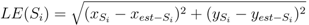The parameters used in the simulation study are summarized in Table 1.
Table 1. Simulation Parameters.
Table 1. Simulation Parameters.
ParameterValue
Number of Sensor Nodes, N150/200/250/300/350/400
Beacon Ratio0.1–0.3
Sensor Field Size50 m× 50 m
Transmission Range of a Sensor Node, r10 m
Number of Component NN, K7

4.1. Performance Comparison

The performance of the proposed LNNE system is compared with two well-known range-free localization algorithms, Centroid and DV-Hop, as well as the localization algorithm based on signal neural network, LSNN.
Figure 3. Sample network with 400 sensor nodes and a beacon ratio of 0.2.
Figure 3. Sample network with 400 sensor nodes and a beacon ratio of 0.2.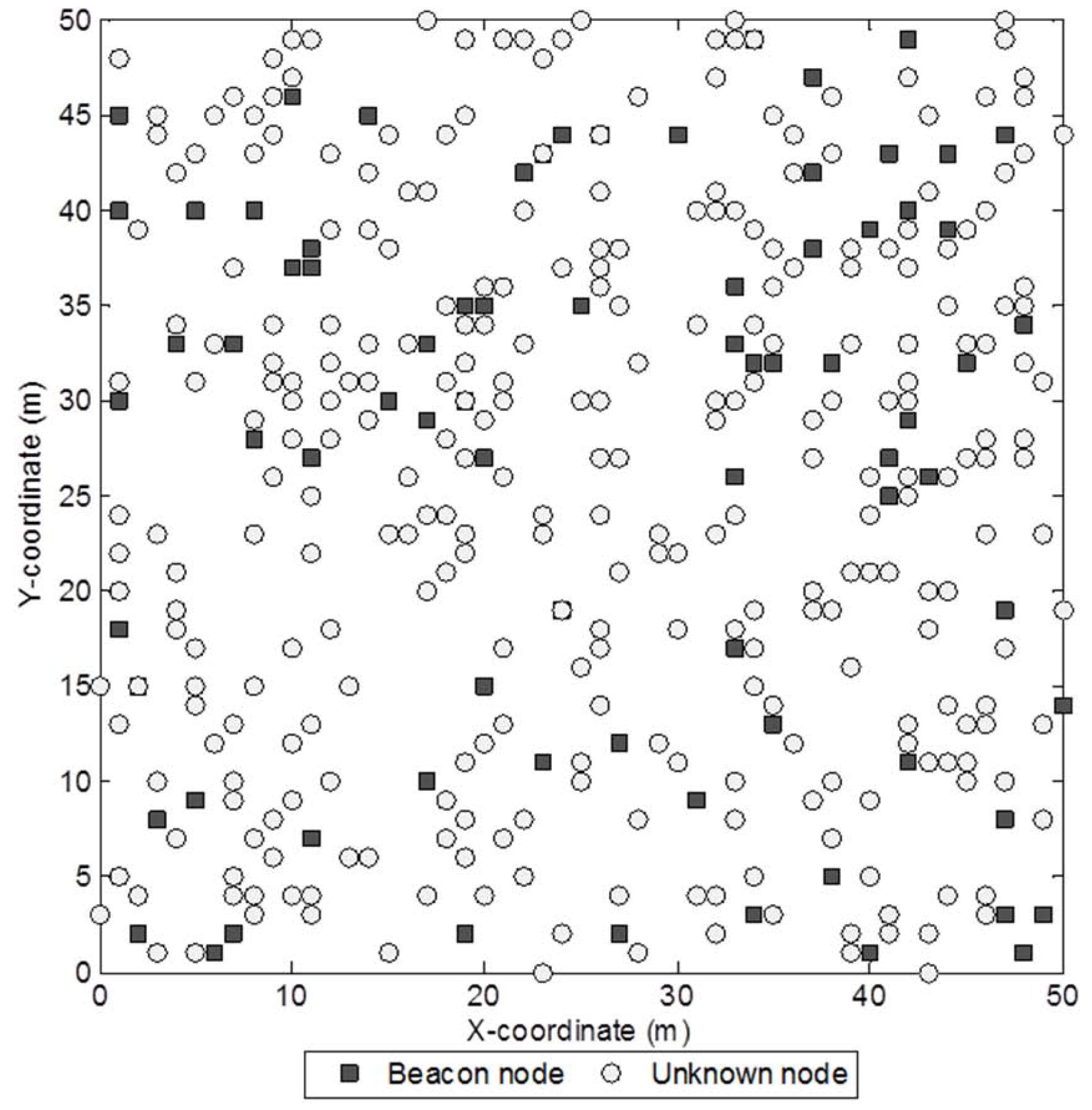4.1.1. Effects of Beacon Ratio and Network Density

In the first two studies, the sensor nodes are uniformly placed in the field without coverage hole. Figure 3 shows a sample network with 400 sensor nodes and a beacon ratio of 0.2. We first investigate the effect of beacon ratio on the performance of the four localization algorithms. The number of sensor nodes in the field is set to 250. The number of beacon nodes varies from 25 to 75, which corresponds to a beacon ratio of 0.1 to 0.3. The simulation results are shown in Figure 4. Generally, the localization errors of the four algorithms are decreased with the increment of beacon ratio. The proposed LNNE outperforms other three algorithms in all cases. For LNNE, the two combination rules, mean rule and median rule, produce comparable results. Compared with LSNN, LNNE can improve the localization accuracy up to 20.7% as shown in Figure 5.
Next, the effect of network density on the performance of the localization algorithms is studied. The network density is changed by varying the number of sensor nodes in the field. In the simulation, the number of sensor nodes, N , varies from 150 to 400 while the beacon ratio is fixed at 0.2. Figure 6 shows the simulation results. Better localization accuracy can be observed for a denser network. For all cases, the proposed LNNE has the best performance. Similar to the previous experiment, the mean rule and median rule achieve comparable results for LNNE. As shown in Figure 7, LNNE significantly improves the localization accuracy compared with LSNN with an average improvement of more than 20%.
Figure 4. Performance comparison among different beacon ratios (N =250, beacon ratio=0.1–0.3, no coverage holes).
Figure 4. Performance comparison among different beacon ratios (N =250, beacon ratio=0.1–0.3, no coverage holes).Figure 5. Performance improvement of LNNE vs. LSSN (N = 250, beacon ratio = 0.1–0.3, no coverage holes).
Figure 5. Performance improvement of LNNE vs. LSSN (N = 250, beacon ratio = 0.1–0.3, no coverage holes).Figure 6. Performance comparison among different network densities (N = 150 to 400, beacon ratio = 0.2, nocoverage holes).
Figure 6. Performance comparison among different network densities (N = 150 to 400, beacon ratio = 0.2, nocoverage holes).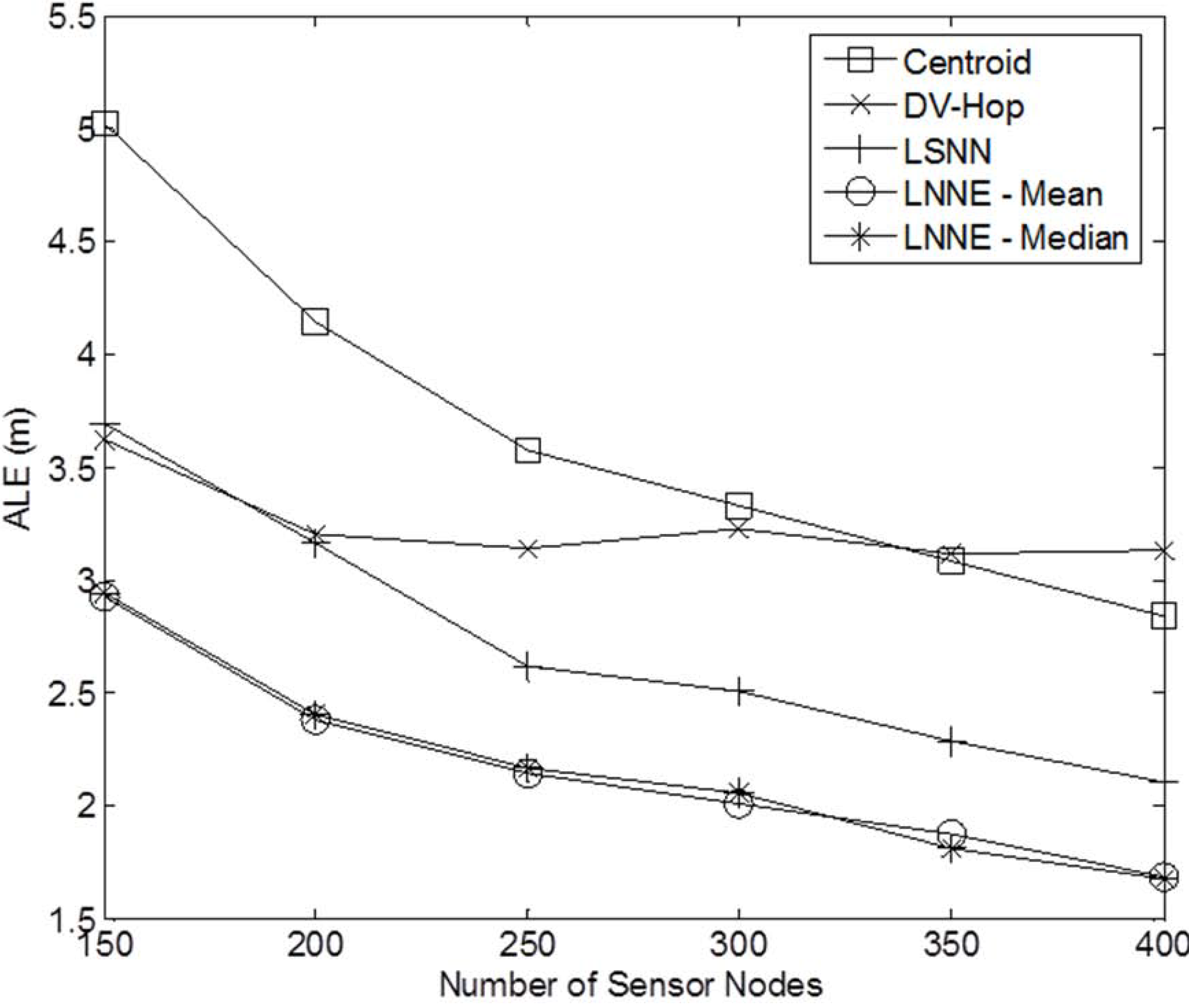Figure 7. Performance improvement of LNNE vs. LSSN under different network densities (N = 150 to 400, beacon ratio = 0.2, no coverage holes).
Figure 7. Performance improvement of LNNE vs. LSSN under different network densities (N = 150 to 400, beacon ratio = 0.2, no coverage holes).4.1.2. Effects of Coverage Holes

Due to the obstacles in the sensor field, there are normally coverage holes in the sensor network. Similar to [15,17], we consider two cases: (1) one circular hole centered at (25, 25) with the radius of 10m; (2) five circular holes with the radius of 5m centered at (25, 25), (10, 10), (10, 40), (40, 10), and (40, 40), respectively. In this experiment, we consider networks with 250 sensor nodes and the beacon ratio varying from 0.1 to 0.3.Sample networks for the two cases with a beacon ratio of 0.2 are shown in Figure 8.
Figure 9 and Figure 10 show the performance of the four localization algorithms for the two coverage hole cases, respectively. Similar to the case of no coverage hole in the network, LNNE achieves significantly better performance than other three localization algorithms. The two combination rules of LNNE produce comparable results. The effect of the coverage holes on the performance of LNNE (median rule) is shown in Figure 11. It can be observed that the performance of LNNE degrades in most cases because of the coverage holes, especially in the cases that a large coverage hole (obstacle) in the network or the beacon ratio is low. However, LNNE still maintains high localization accuracy as shown Figure 9 and Figure 10.
Figure 8. Sample networks with coverage holes (250 sensor nodes and 50 beacon nodes):(a) 1 hole; (b) 5 holes.
Figure 8. Sample networks with coverage holes (250 sensor nodes and 50 beacon nodes):(a) 1 hole; (b) 5 holes.Figure 9. Performance comparison under different beacon ratios (0.1-0.3) and 1 coverage hole.
Figure 9. Performance comparison under different beacon ratios (0.1-0.3) and 1 coverage hole.Figure 10. Performance comparison under different beacon ratios (0.1-0.3) and 5 coverage holes.
Figure 10. Performance comparison under different beacon ratios (0.1-0.3) and 5 coverage holes.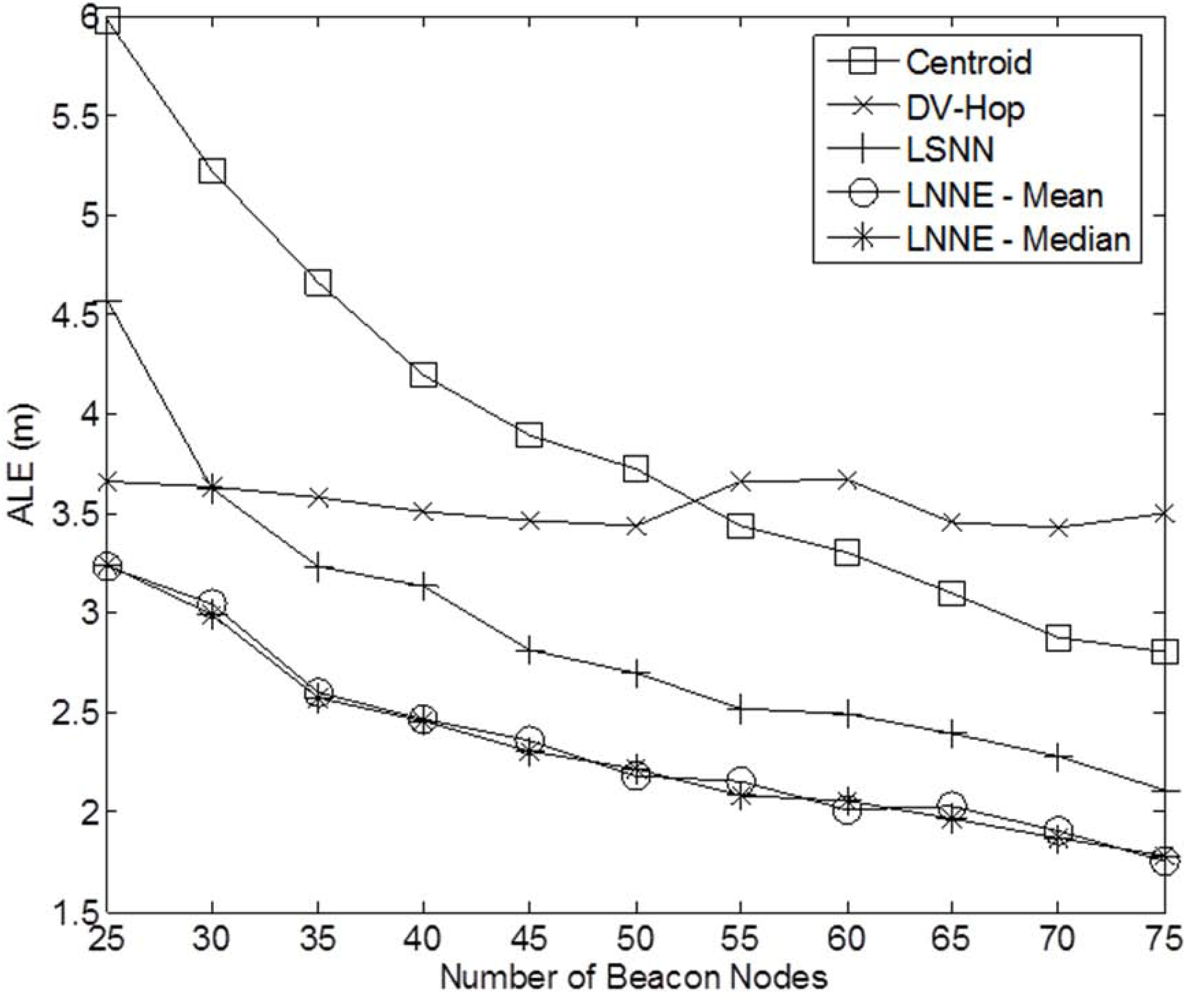Figure 11. Effect of coverage holes on LNNE (N = 250, beacon ratio = 0.1-0.3).
Figure 11. Effect of coverage holes on LNNE (N = 250, beacon ratio = 0.1-0.3).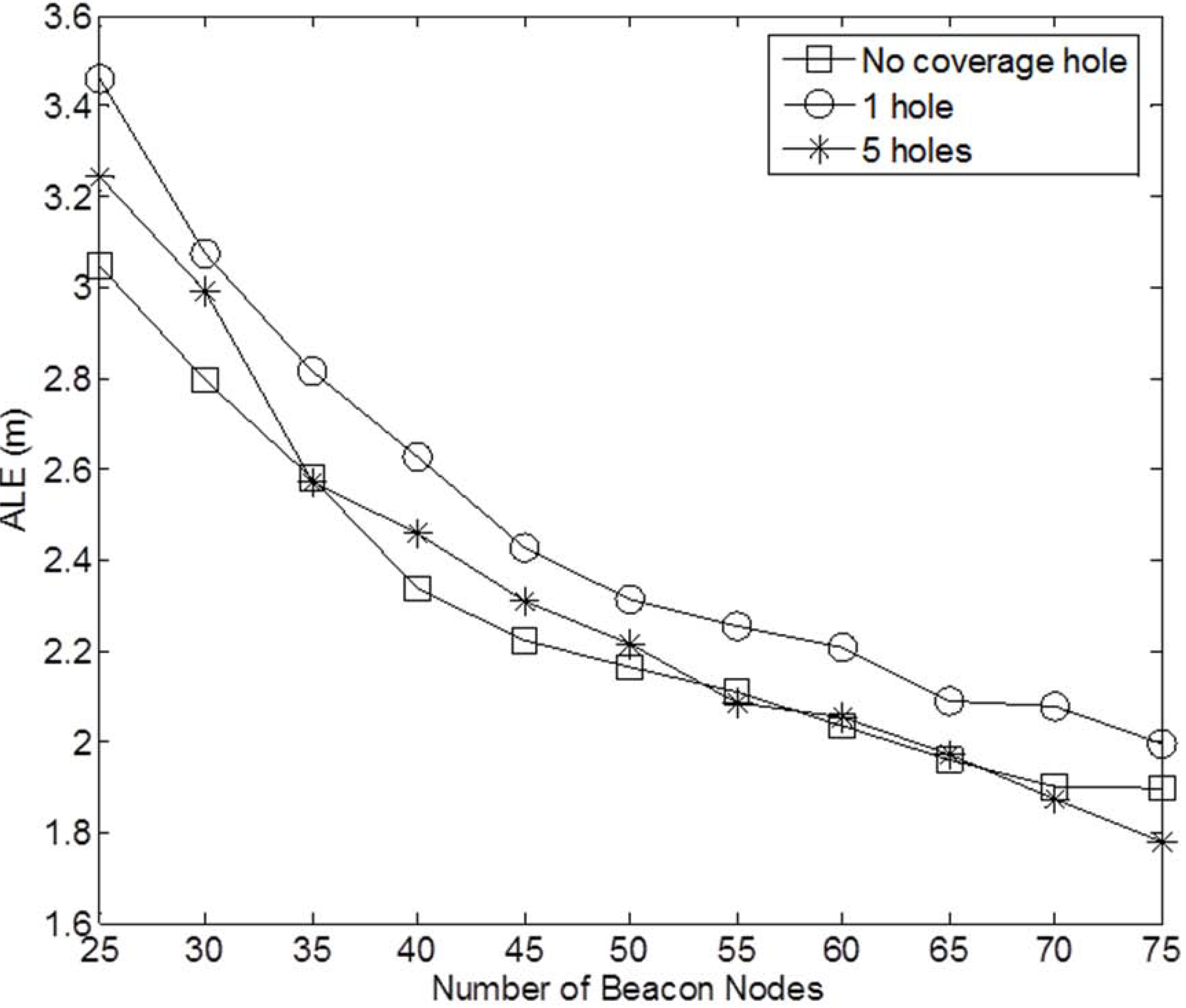Figure 12. Performance of refinement algorithms under different beacon ratios (N = 250, beacon ratio = 0.1-0.3, no coverage holes).
Figure 12. Performance of refinement algorithms under different beacon ratios (N = 250, beacon ratio = 0.1-0.3, no coverage holes).4.2. Improving Localization Accuracy with EMSO Algorithm

The performance of the refinement algorithms is also investigated. We study the effect of MMSO and EMSO on the performance of LSNN and LNNE. Only median rule is considered for LNNE since the mean rule produces comparable result as shown in Section 4.1. The maximum number of iterations for MMSO and EMSO is 100. The simulation results for networks without coverage holes are shown in Figure 12 and Figure 13. The results demonstrate that MMSO can significantly improve the performance of LSNN and LNNE. EMSO can further improve the localization accuracy by utilizing the location information of the neighboring beacon and unknown nodes. The combination of LNNE and EMSO consistently achieves the best performance. With EMSO, the performance of LNNE can be improved by 24% on average, compared with an average improvement of 11% by MMSO.
Figure 13. Performance of refinement algorithms with different network densities (N = 150 to 400, beacon ratio = 0.2, no coverage holes).
Figure 13. Performance of refinement algorithms with different network densities (N = 150 to 400, beacon ratio = 0.2, no coverage holes).5. Conclusions

In this paper, neural network ensembles are used to improve the localization accuracy for WSNs by utilizing the diversity of the component neural networks. The localization system, LNNE, only utilize the connectivity information of the network to estimate the location of sensor nodes. Simulation studies are carried out to compare the performance of LNNE with two well-known range-free localization algorithms, Centroid and DV-Hop, and a single neural network-based localization algorithm, LSNN. The effects of beacon ratio, network density, and coverage holes on the performance of LNNE are investigated. The experimental results demonstrate that LNNE outperforms other three algorithms in all simulation cases. The EMSO algorithm is also proposed, which can significantly improve the performance of LNNE with the help of location information of the neighboring beacon and unknown nodes.

Acknowledgments

The work of Ashgar Dehghani was supported by Petroleum Recovery Research Center (PRRC) of New Mexico Institute of Mining and Technology.

References

1. Akyildiz, I.; Su, W.; Sankarasubramaniam, Y.; Cayirci, E. Wireless sensor networks: A survey. Comput. Netw. 2002, 38, 393–422. [Google Scholar] [CrossRef]
2. Kunz, T.; Tatham, B. Localization in wireless sensor networks and anchor placement. J. Sens. Actuator Netw. 2012, 1, 36–58. [Google Scholar]
3. Tarrio, P.; Bernardos, A.M.; Casar, J.R. Weighted least squares techniques for improved received signal strength based localization. Sensors 2011, 11, 8569–8592. [Google Scholar] [CrossRef]
4. Whitehouse, C. The design of calamari: An ad hoc Localization System for Sensor Networks. M.Sc. Thesis, University of California at Berkeley, Berkeley, CA, USA, 2002. [Google Scholar]
5. Wen, C.-Y.; Chen, F.-K. Adaptive AOA-aided TOA self-positioning for mobile wireless sensor networks. Sensors 2010, 10, 9742–9770. [Google Scholar] [CrossRef]
6. Kwon, Y.; Mechitov, K.; Sundresh, S.; Kim, W.; Agha, G. Resilient Localization for Sensor Networks in Outdoor Environments, Technical Report, University of Illinois at Urbana-Champaign: Champaign, IL, USA, 2004.
7. Priyantha, N. The Cricket Indoor Location System. Ph.D. Dissertation, Massachussette Institute of Technology, Cambridge, MA, USA, 2005. [Google Scholar]
8. Niculescu, D.; Nath, B. Ad Hoc Positioning System (aps) Using Aoa. In Proceedings of the IEEE Infocom 2003, San Francisco, CA, USA, 30 March–3 April 2003.
9. Priyantha, N.; Miu, A.; Balakrishnan, H.; Teller, S. The Cricket Compass for Context-aware Mobile Applications. In Proceedings of the ACM Mobicom 2001, Rome, Italy, 16–21 July 2001.
10. Bulusu, N.; Heidemann, J.; Estrin, D. Gps-less low cost out-door localization for very small devices. IEEE Pers. Commun. Mag. 2000, 7, 28–34. [Google Scholar]
11. He, T.; Huang, C.; Blum, B.; Stankovic, J.A.; Abdelzaher, T. Range-Free Localization Schemes in Large Scale Sensor Networks. In Proceedings of the ACM Mobicom 2003, San Diego, CA, USA, 14–19 September 2003.
12. Lazos, L.; Poovendran, R. SeRLoc: Secure Range-independent Localization for Wireless Sensor Networks. In Proceedings of the ACM WiSe 2004, Philadelpia, PA, USA, 26 September–1 October 2004.
13. Shang, Y.; Ruml, W.; Zhang, Y.; Fromherz, M.P. Localization from Mere Connectivity. In Proceedings of the ACM Mobihoc 2003, Annapolis, MD, USA, 1–3 June 2003.
14. Niculescu, D.; Nath, B. Ad-hoc Positioning Systems. In Proceedings of GLOBECOM’01, San Antonio, TX, USA, 25–29 November 2001; pp. 2926–2931.
15. Chatterjee, A. A Fletcher-Reeves conjugate gradient neural-network-based localization algorithm for wireless sensor networks. IEEE Trans. Veh. Technol. 2010, 59, 823–830. [Google Scholar] [CrossRef]
16. Nguyen, X.; Jordan, M.I.; Sinopoli, B. A kernel-based learning approach to ad hoc sensor network localization. ACM Trans. Sens. Netw. 2005, 1, 134–152. [Google Scholar] [CrossRef]
17. Tran, D.A.; Nguyen, T. Localization in wireless sensor networks based on support vector machines. IEEE Trans. Parallel Distrib. Syst. 2008, 19, 981–994. [Google Scholar] [CrossRef]
18. Yun, S.; Lee, J.; Chung, W.; Kim, E.; Kim, S. A soft computing approach for localization in wireless sensor networks. Expert Syst. Appl. 2009, 36, 7552–7561. [Google Scholar] [CrossRef]
19. Shilton, A.; Sundaram, B.; Palaniswami, M. Ad-hoc Wireless Sensor Network Localization Using Support Vector Regression. In Proceedings of the ICT Mobile Summit, Stockholm, Sweden, 10–12 June 2008.
20. Krogh, A.; Sollich, P. Statistical mechanics of ensemble learning. Phys. Rev. E 1997, 55, 811–825. [Google Scholar] [CrossRef]
21. Wichard, J.D.; Ogorzalek, M. Time series predication with ensemble models applied to the CATS benchmark. Neurocomputing 2007, 70, 2371–2378. [Google Scholar]
22. Zheng, J. Predicting software reliability with neural network ensembles. Expert Syst. Appl. 2009, 36, 2166–2122. [Google Scholar]
23. Fletcher, R.; Reeves, C.M. Function minimization by conjugate gradients. Comput. J. 1964, 7, 149–154. [Google Scholar] [CrossRef]
24. Priyantha, N.; Balakrishman, H.; Demaine, E.; Teller, S. Anchor-free Distributed Localization in Sensor Networks. In Proceedings of the ACM Sensys 2003, Los Angeles, CA, USA, 5–7 November 2003.